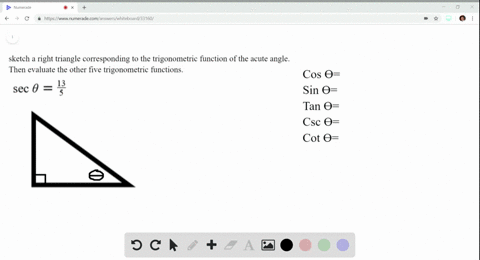Enroll in one of our FREE online STEM summer camps. Space is limited so join now!View Summer Courses### Evaluating Trigonometric Functions In Exercises $… 03:05University of California, Berkeley Need more help? Fill out this quick form to get professional live tutoring. Get live tutoring Problem 19 Evaluating Trigonometric Functions In Exercises$17-20$, sketch a right triangle corresponding to the trigonometric function of the acute angle$\theta$. Then evaluate the other five trigonometric functions of$\theta\$.
$$\cos \theta=\frac{4}{5}$$

## Discussion

You must be signed in to discuss.

## Video Transcript

Okay, so we're given coastline of data, is good for over five and were asked to find the rest of the angles. Well, we know that speaking of data, just the reciprocal of Cosette, a bitter set up five over four and for the very meaning we need to find our right triangle between a close, an idea is opposite or not upset. Jason set up, pour over hypotheses. So we need to find the opposite side bar angle. So it seems you've got a degree in dim that's r squared is equal to x squared plus y squared. So we have X is four squared plus y squared is because our square, which is five squared. That's 25 minus 16 people to wife Quite. Which gives me dad the square root of nine. Because wine and why is equal to okay, Okay, you know we have there beside, so it Look for a sign and beta, It's all over. Pardon me, Canyon. A video which is opposite over adjacent coach engine of data. The reciprocal tangent. That's for three. And co Speaking of data, which is typical of signs which is five over three